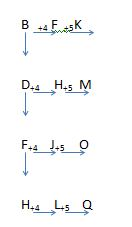# SSC CGL 2018 Practice Test Papers | Reasoning Ability (Day-14)

Dear Aspirants, Here we have given the Important SSC CGL Exam 2018 Practice Test Papers. Candidates those who are preparing for SSC CGL 2018 can practice these questions to get more confidence to Crack SSC CGL 2018 Examination.

[WpProQuiz 2852]

Click “Start Quiz” to attend these Questions and view Explanation

1). In the following questions, select the related letters from the given alternatives.

COLOSSAL: ENORMOUS:: GRUFF : ?

1. IMPORTANT
2. SORROW
3. ROUGH
4. RESPECT

2). In the following questions, select the related letters from the given alternatives.

33:1095: 23: ?

1. 534
2. b.589
3. 689
4. 1000

3). ‘Chicken pox’ is related to ‘Virus’ in the same way ‘Malaria’ is related to

1. Algae
2. Protozoa
3. Virus
4. Mosquito

4). Find the odd one.

1. 12 – 30
2. 24 – 42
3. 56 – 74
4. 33 – 52

5). Arrange the given words in the sequence in which they occur in the dictionary.

1. Came
2. Come
3. Camel
4. Common
1. i, iii, ii, iv
2. iv, iii,I , ii
3. iii, ii, iv, i
4. ii, iv, I, iii

6). A series is given with one term missing. Select the correct alternative from the given ones that will complete the series.

BFK, DHM, FJO ?

1. ILM
2. HLQ
3. JPQ
4. LMO

7). Six boxes are kept one on top of the other. Box 1 is just above Box 3. The Box 6 is between Box 5 and Box 2. Box 4 is between Box 1 and Box 5. Which box is between the Box 4 and Box 6 games?

1. Box 2
2. Box 6
3. Box 1
4. Box 5

8). Six boxes are kept one on top of the other. Box 1 is just above Box 3. The Box 6 is between Box 5 and Box 2. Box 4 is between Box 1 and Box 5. Which box is placed in the topmost position?

1. Box 2
2. Box 6
3. Box 1
4. Box 5

9). Anand ranks 6th from the bottom and 28th from the top in a class. How many students are there in the class?

1. 31
2. 35
3. 32
4. 33

10). In the following question, correct the equation by interchanging two signs.

5 + 7÷21×3−3 = 5

1. ÷ and –
2. × and +
3. × and =
4. = and +

11. In the following question, select the word which cannot be formed using the letters of the given word.

MARVELOUS

1. Seal
2. Male
3. Rust
4. Mars

Enormous is the synonym of colossal. Rough is the synonym of GRUFF.

33×33+3+3=1095

23×23+2+3=534

Chicken pox’ is caused by ‘Virus’ whereas Malaria is caused by ‘Protozoa’.

12+18=30

24+18=42

56+18=74

33+19=52

All other options are in multiples of 3 except 52

Came, camel, come, commonThe arrangement is:

Box 2

Box 6

Box 5

Box 4

Box 1

Box 3

1. Box 2
2. Box 6
3. Box 1
4. Box 5

Total students = 6 + 28 – 1 = 33